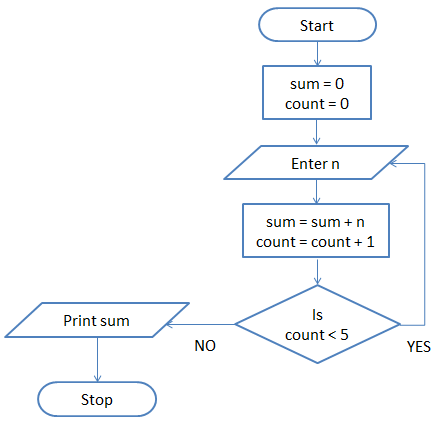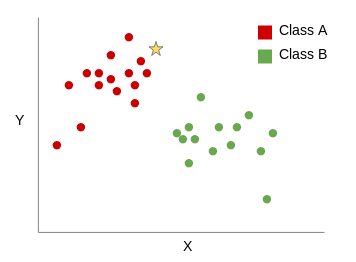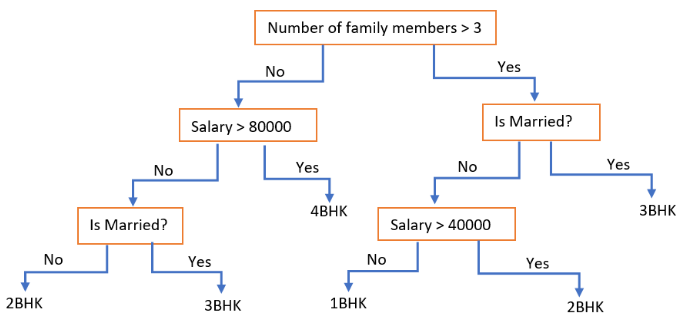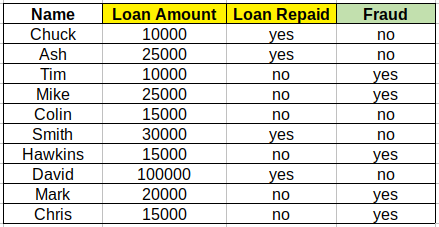Home » Applied Machine Learning Assessment Test

# Applied Machine Learning Assessment Test

How will you assign 4 to a variable ‘num’ and ‘Robin’ to another variable ‘name’?

Select the right code to get the following output:

p

y

t

h

o
n

if age is less than 5 :

output is “A”

else if age is less than 50 :

if age if less than 15 :

output is “B”

else :

output is “C”

else :

output is “D”

Study the following flowchart and select the correct option.In machine learning, what does classification mean?

Given below is a scatter plot of two numerical variables X and Y. A new data point, denoted by the star symbol, is likely to belong to which of the two classes.What will be the output of the following flowchart for a married person with a family size of 3 and earning less than 80,000?Given below is a loan dataset with customer name, loan amount, loan repayment status and fraud status as its attributes. Which attribute is more important to determine the fraud status?A data scientist is trying to build a model to classify images as either containing a cat and dog. He built 3 models for this task. The details of these models’ performance are given below:

Model 1

• Correctly classified 75% of the images containing a cat.

• Correctly classified 45% of the images containing a dog.

Model 2

• Correctly classified 30% of the images containing a cat.

• Correctly classified 60% of the images containing a dog.

Model 3

• Correctly classified 80% of the images containing a cat.

• Correctly classified 22% of the images containing a dog.

Which model combination, if used together, will correctly classify at least 50% of the images containing a cat and a dog, respectively?

Let’s say you want to build a predictive model to detect if a patient has a certain disease or not. You have decided to evaluate your model based on the accuracy score, i.e., ratio of number of correctly predicted cases and the total number of cases in the dataset.

What should be the proportion of the target classes in the data used to build the predictive model, give the evaluation metric is accuracy?

A data scientist has developed a breast cancer classification model. He wants to predict if the cancer diagnosis is benign or malignant based on several features. He wants to test his model on a dataset of 10,000 observations with 97% of them as benign and the rest as malignant.

What should be the expectations from his model? Select the correct option.

A variable x contains grades of students from a college. The unique values of x are A, B, C, and D. Select the correct option.

Select the code which will give the output “Hello World”:

Consider the python list below:

A = [23, 58, 49, 99, 120, 256, 1024]

What is the result of A – A ?

How can we add a element ‘Jim’ to the list ‘names’?

names = [233, ‘Paul’, ‘A34’]

What will be the output of the code below?

dict = {‘p’:2,’q’:5,’r’:8}

print(dict[‘q’])

What will be the output of the variable ‘sum’ in the following code?

num = [3,6,1,7,8]

sum = 0

for i in num:

sum+= i

What will be the output of the code below?

import numpy as np
np.arange(10)

Create a dataframe from the dictionary below:

dict ={‘a’:[5,2,8], ‘b’:[8,4,2], ‘c’:[3,4,5]}

How will you find the sum of every row in the dataframe below?

Import pandas as pd

df = pd.DataFrame({‘a’: [20,30,40], ‘b’: [200, 300, 400]})

Thank you for taking our survey.